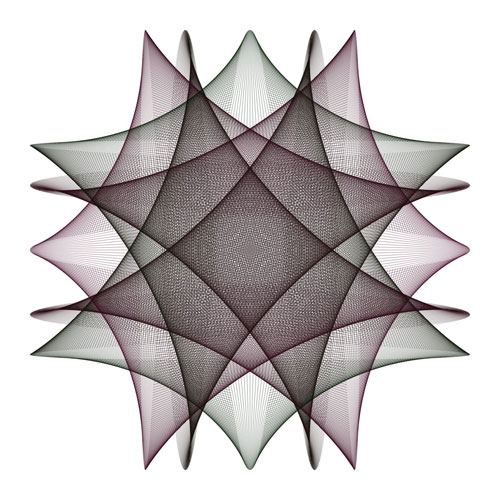Math Central - mathcentral.uregina.caMath Beyond Schoolreturn to topThe art of Hamid Naderi Yeganeh Art and Math may at first seem to be very differing things, but people who enjoy math tend to look for mathematics in art.  They want to see the patterns and angles and lines of perspective.  This is why artists like M.C. Escher appeal to mathematicians so much.  There is a large amount of math involved in art, not to mention basic things like measuring and lines, but the intricacies of art can often be described using math.This image shows 2,000 line segments. For each i=1,2,3,...,2000 the endpoints of the i-th line segment are: $\left( \sin\left( \frac{10 \pi i}{2000}\right),\; \frac12 \cos\left( \frac{2 \pi i}{2000}\right) \right)$ and $\left( \frac12 \sin \left( \frac{2 \pi i}{2000}\right),\; \cos \left( \frac{10 \pi i}{2000}\right) \right).$ Other works of Hamid Naderi Yeganeh can be found at
 Math Central is supported by the University of Regina and The Pacific Institute for the Mathematical Sciences.about math central :: site map :: links :: notre site français